#### You may also like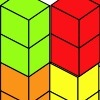### Triple Cubes

This challenge involves eight three-cube models made from interlocking cubes. Investigate different ways of putting the models together then compare your constructions.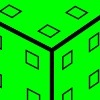### Inky Cube

This cube has ink on each face which leaves marks on paper as it is rolled. Can you work out what is on each face and the route it has taken?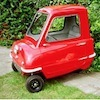### The Car That Passes

What statements can you make about the car that passes the school gates at 11am on Monday? How will you come up with statements and test your ideas?

##### Age 7 to 11 Challenge Level:

This is your chance to follow some numbers and see where they go!

A simple rule is all you need.
My first suggestion is to add the digits together then multiply (times) by $2$.
The first number that I chose happened to be $56$.

So, let's start:

We add the $5$ and $6$, $5+6=11$
We multiply the $11$ by $2$, $2 \times 11 = 22$, and that's the first part of the journey.

So, where now?
We carry on with this rule:

We add the $2$ and $2$,  $2+2=4$
We multiply the $4$ by $2$,  $4 \times 2 = 8$, and that's the second part the journey.

Now, $8 + 0 = 8$ and $8 \times 2 = 16$ and that was the third part.

And, $1 + 6= 7$, and $7 \times 2 = 14$

Do the same to $14$ and we get $10$

$10$ leads to $2$, $2$ leads to $4$, $4$ leads to $8$ and we are back to where we got to in the second part of the journey.

If we went on and on and wrote down where we got to after each part we would see something like:
$56, 22, 8, 16, 14, 10, 2, 4, 8, 16, 14, 10, 2, 4, 8, ...$

After exploring that journey it's time to start somewhere new, for example $11$ which goes along like this;:

$11, 4, 8, 16, 14, 10, 2, 4, 8, ...$

Oh! So we are on the same bit as before, a circular bit that goes $2, 4, 8, 16, 14, 10$ and then back to the $2$ again.

Now a new starting place, $96$.
This goes like this:

$96, 30, 6, 12, 6, 12, 6, ...$

Oh! So we now have a smaller circular bit of the journey that goes $6, 12$ then back to the $6$.

I explored further trying to start with each number from $1$ to $99$.

Then I tried similar, but different rules.
I found I needed a big piece of plain paper and used arrows to show the journeys.  Here are just some bits of them to tempt you to go further: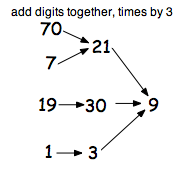and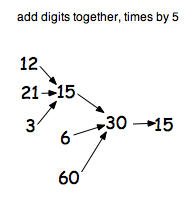There are $99$ starting points to try and I've only show you $8$ on each of the two above so there are lots more to explore!

Decide on the rules you will use and investigate what happens with different starting points.